# Metric projection

(diff) ← Older revision | Latest revision (diff) | Newer revision → (diff)

operator of best approximation

A many-valued mapping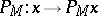, associating to each elementof a metric spacethe setof elements of best approximation (cf. Element of best approximation) from the set. Ifis a Chebyshev set, then the metric projection is a single-valued mapping. The problem of constructing an element of best approximation is often solved approximately, that is, an element is determined in the setwhereis sufficiently small. From the properties of the mapping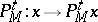it is sometimes possible to obtain properties of the set. E.g., if for any elementof a normed spacea number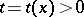exists such thatis convex (connected), thenis convex (respectively, connected).

From the point of view of applications it is useful to know whether the metric projection has such properties as linearity, continuity, uniform continuity, etc. A metric projection on a Chebyshev subspace of a normed space is, in general, not linear. If the metric projection on each subspace of fixed dimension is single-valued and linear, thenis linearly isometric to an inner-product space. The metric projection on a non-empty approximately-compact set in a metric space is upper semi-continuous; in particular, in a normed space the metric projection onto a finite-dimensional Chebyshev subspace is continuous; the metric projection may be not lower semi-continuous if the subspace is not Chebyshev. There exists a reflexive strictly-convex space and an infinite-dimensional subspace on which the metric projection is discontinuous. The metric projection on any closed convex setin a Hilbert space satisfies a Lipschitz condition: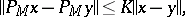with constant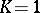.

The continuity property of a metric projection and its generalizations have found applications in ill-posed problems, in the convexity problem for Chebyshev sets, in the construction of elements of best approximation, etc.

How to Cite This Entry:
Metric projection. Encyclopedia of Mathematics. URL: http://encyclopediaofmath.org/index.php?title=Metric_projection&oldid=13496
This article was adapted from an original article by V.I. Berdyshev (originator), which appeared in Encyclopedia of Mathematics - ISBN 1402006098. See original article